### Number of Paths in Rectangular Grid

Source: Solved it during Algorithms Course under Prof Diwan, and discussed with Nikhil Jain (IT BHU 2008 Alumnus, Product Manager at AskLaila)

Problem:

1. (Easy)

Consider a n x m rectangular grid. The problem is to find the number of shortest (or monotonic in this case) paths along the edges of the cell that start at (0,0) and end at (n,m).

A monotonic path is a path always moving towards the goal, i.e. consists of moving up or right but not down or left. Figure 1 illustrates such a path for a grid of size 5 x 3.

2. (Difficult)

Now we need to print all monotonic paths that do not go above the diagonal y = x. Figure 2 shows such a path. Note that the path in figure 1 goes above the diagonal, hence not desirable in this case. How many such paths exist for n x m grid (n >= m)?

Disclaimer:
The first problem is too simple, and the second is very challenging. Have fun :-)

1.8C3 or 8C5 = 56 i.e., (n+m)Cn for the first one. Still figuring the second one.

2.first problem which is too simple :
static int counter;
int noOfRows = 3;
int noOfColumns = 3;
int noOfPaths = numberOfWays(noOfRows, noOfColumns, 0, 0)

public static int numberOfWays(int rows, int cols, int row, int col){
if(row > rows || col > cols) return 0;
if(row == rows && col == cols){
counter++;
}
numberOfWays(rows, cols, row+1, col);
numberOfWays(rows, cols, row, col+1);
return counter;
}

3.For second problem simply adding one more condition as suggested in the question itself should do:

//counter is static variable
static int counter;
int noOfRows = 3;
int noOfColumns = 3;
int noOfPaths = numberOfWays(noOfRows, noOfColumns, 0, 0)

public static int numberOfWays(int rows, int cols, int row, int col){
if(row > rows || col > cols) return 0;
if(! (row>=col)) return 0; //--> this has been //added.
if(row == rows && col == cols){
counter++;
}
numberOfWays(rows, cols, row+1, col);
numberOfWays(rows, cols, row, col+1);
return counter;
}

Solving this problem in non-recursive call would be interesting.

4.For the difficult puzzle: If m=n, the answer is Catalan numbers. Maybe it's possible to generalize that proof idea for m!=n?

5.1) 56
2) by first eliminating the left most row and converting the problem to 4*3 since no steps can be on left most edge and than subtracting the rest of ways involving steps above the diagonal: 27

6.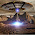Let W(m,n) be the total number of ways of going from point (0,0) to (m,n) monotonically.

Solution to part 1

Let S(0,n) = 1. (for all n)

Then S(l,n) = Summation from k=1 to k=n (S(l-1,k)).

ie. S(1,n) = n.

S(2,n) = n(n+1)/2.

and so on..

Then W(m,n) = Summation from l=0 to l=m (S(l,n)).

Solution to part 2

If W'(m,n) be total no. of ways then

W'(m,n) = W(m,n) - no. of paths which lie above the diagonal - no. of paths which cut the diagonal at (1,1) while going towards north - no. of paths which cut the diagonal at (2,2) while going towards north - and so on.

W'(m,n) = W(m,n) - summation from p=0 to p=m-1 (W(p,n-m+2+p)).

7.The second part of my first post was wrong..

Let W(m,n) be the total number of ways of going from point (0,0) to (m,n) monotonically.

Solution to part 1

Let S(0,n) = 1. (for all n)

Then S(l,n) = Summation from k=1 to k=n (S(l-1,k)).

ie. S(1,n) = n.

S(2,n) = n(n+1)/2.

and so on..

Then W(m,n) = Summation from l=0 to l=m (S(l,n)).

Solution to part 2

If W'(m,n) be total no. of ways in which the path doesn't go above the diagonal, then

W'(m,n) = W(m,n) - no. of paths which starts above the diagonal - no. of paths which cut the diagonal at (1,1) while going towards north - no. of paths which cut the diagonal at (2,2) while going towards north - and so on.

W'(m,n) = W(m,n) - summation from p=0 to p=m-1 (W(p,n-m+1+p)).

8.Again there is a mistake in my second part :P

W'(m,n) = W(m,n) - summation from p=0 to p=m-1 [f(p)x(W(p,n-m+1+p))].

where f(m-1) = f(m-2) = 1

and f(p) = 2f(p+1) +1.

For (3,5) the answer comes out to be 56 and 28.

Also W(m,n) = (m+n)Cn.

9.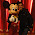I guess the answer to the 2nd part is 47.
Let i denote the row number and j denote the column number
Let f(i, j) denote the count of possible number of options to go in the (i+1)th row from the point (i,j) after MANDATORY 1st STEP IN THE NORTH. So,

Correct Options = f(0,0) + f(0,1) + f(0,2) + f(0,3) + f(0,4) + f(0,5)

Now, f(0,0) = 0 since we can't go north on the first step.
Now, we can go to points (1,1), (1,2)...(1,5) from (1,1), but not on (0,0) because then we have to take a step back.
So,
f(0,1) = f(1,1) + f(1,2) + f(1,3) + f(1,4) + f(1,5)
Similarly,
f(0,2) = f(1,2) + f(1,3) + f(1,4) + f(1,5)
f(0,3) = f(1,3) + f(1,4) + f(1,5)
f(0,4) = f(1,4) + f(1,5)
f(0,5) = f(1,5)

So Correct Options = 0 + f(1,1) + 2f(1,2) + 3f(1,3) + 4f(1,4) + 5f(1,5)
= f(1,1) + 2f(1,2) + 3f(1,3) + 4f(1,4) + 5f(1,5)
On the same lines,
f(1,1) = 0
f(1,2) = f(2,2) + f(2,3) + f(2,4) + f(2,5)
f(1,3) = f(2,3) + f(2,4) + f(2,5)
f(1,4) = f(2,4) + f(2,5)
f(1,5) = f(2,5)

So Correct Options = 0 + 2f(2,2) + 5f(2,3) + 9f(2,4) + 9f(2,5)

Now f(2,2) = 0
And the points (2,3), (2,4) and (2,5) can be treated as normal points since there is no restriction on any three of them as y=x line doesn't interfere for any of these points now.
So,
f(2,3) = 3C1 = 3
f(2,4) = 2C1 = 2
f(2,5) = 1C1 = 1

So, Correct options = 0 + 5*3 + 9*2 + 14*1
= 47

10.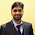The answer to second part is 28.
E(m,n)= no. of ways of reaching coordinate m,n.
E(m,n) =E(m-1,n)+E(m,n-1).
base cases
E(x,0)=1;
E(x,x)=E(x,x-1);

Running time using dynamic programming is O(mn).

11.To reach the destination let us consider our journey along y direction. since it is a n*m field we could divide the y journey into a max of n+1 different steps y1,y2,y3...
and proceed as follows:

Answer to the first part is number of solutions to the equation y1+y2+y3.....yn+1=m with the condition that yi>=0. It is the coefficient x^m in (1+x+x^2....x^m)^n+1 which is (n+m)Cm.
in part 2: n>m
for the second part there are extra constraints on y1 to ym such that y1<=0 y2<=1 y3<=2.....ym<=m-1.
number of ways is the coefficent of x^m in 1*(1+x)*(1+x+x^2)....*(1+x+x^2+....x^(m-1))*((1+x+x^2..x^m)^(n-m+1)).
I am unable to figure out how to evaluate the coefficient in the above expression by using binomial expansions( infinite series formulae) .

If the above thing works we could have a general formula.

12.First Part is trivial. Ans : (m+n)Cm

Second part is tricky. Use principal of Reflection to solve this. Ans : (m+n)Cm - (m+n)C(m-1)
Solution :
Use following graph to visualise this. On the Xnew = X+Y, and Ynew as X-Y.
Every step in new axis is 45 degree upward or 45 degree downward. And constraint will be that no point should touch Y=-1 line. Aim is to find #paths from (0,0) to (n+m, n-m).
Take the reflection of (0,0) in Y=-1 line i.e. (0,-2).
So, #path which touches Y=-1 line will be equivalent to #paths from (0,-2) to (n+m, n-m) (Principle of Reflection)and that will be (m+n)C(m-1).

13.Assumption made in my previous post was that we can allow points on the diagonal but not above that. If we don't even allow points on diagonal then we can start from (1,1) (in new axis) to get total #paths and from (1,-1) to wrong paths.(i.e. taking reflection along line Y=0).
So, solution in this case will be (n+m-1)Cm - (n+m-1)C(m-1).

14.Consider any path from (0,0) to (m,m) which does not go above diagonal. If we add (n-m) horizontal step to this path it will still gives us a path from (0,0) to (n,m) which does not go above diagonal. Conversely, any such path can be represented as a path from (0,0) to (m,m) with (n-m) horizontal steps added to it. Now number of ways in which you can add (n-m) horizontal steps to a path having m horizontal steps and m vertical steps is nCm. We also know that paths from (0,0) to (m,m) which does not go above diagonal are given by well known Catalan number C_m. So total number of paths = nCm * C_m. So I think the answer should be: (nCm)*(2mCm) /(m+1)

15.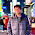second has a classic solution using bijection principle..!! read it in a book

16.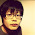1. C(8,3)
2. C(8,3) - C(8,2).

Explanations for 2: flip every step after the first "up" arrow that goes across the diagonal, you will get a bijection to another problem where you will have a 6*2 board, whose solution is C(8,2). Subtract them out is the solution to the original problem.

17.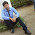ans=56,
in quardinate geometry for (5,3) total moves in x direction is 5 and in y direction 3.
total path is =[ factorial(5+3) ] / [ fac(5) * fac(3) ] = 56

18.for the first part of question -

if you want to go from (0,0) to (n,m) [n horizontal moves and m vertical moves]
we can arrange n 'H' and m 'V' by ((n+m)! / (n)! * (m)!)
here n = 5 and m = 3 so ans is 8C3 = 56

Second part-

We don't want to cross diagonal (0,0) to (3,3) here. In this case we count the faulty cases, where we are crossing that diagonal and remove this from total ((n+m)Cm) to get required answer.

to get faulty cases , we can observe whenever we cross (0,0)->(3,3) diagonal , path will touch or cross diagonal (-1,0)->(2,3).
Now take a part of path ((0,0) to first cut on the diagonal(-1,0)->(2,3)) and reflect it with respect to diagonal (-1,0)->(2,3) . by doing all this, we can observe point (0,0) will map to (-1,-1) and each path from (0,0) to (5,3) will have a corresponding path from (-1,-1) to (5,3)

so faulty cases = number of paths from (-1,-1) to (5,3) is 10C4 (ANSWER)

19.for the first part of question -

if you want to go from (0,0) to (n,m) [n horizontal moves and m vertical moves]
we can arrange n 'H' and m 'V' by ((n+m)! / (n)! * (m)!)
here n = 5 and m = 3 so ans is 8C3 = 56

Second part-

We don't want to cross diagonal (0,0) to (3,3) here. In this case we count the faulty cases, where we are crossing that diagonal and remove this from total ((n+m)Cm) to get required answer.

to get faulty cases , we can observe whenever we cross (0,0)->(3,3) diagonal , path will touch or cross diagonal (-1,0)->(2,3).
Now take a part of path ((0,0) to first cut on the diagonal(-1,0)->(2,3)) and reflect it with respect to diagonal (-1,0)->(2,3) . by doing all this, we can observe point (0,0) will map to (-1,1) and each path from (0,0) to (5,3) will have a corresponding path from (-1,1) to (5,3)

so faulty cases = number of paths from (-1,1) to (5,3) is 8C2
ANSWER = total - faulty = 8C3 - 8C2

By this approach you can find number of paths even for any kind of restricting path like same problem with barrier (0,0) -> (2,2) instead of (0,0) -> (3,3)